International
Tables for
Crystallography
Volume B
Reciprocal space
Edited by U. Shmueli

International Tables for Crystallography (2006). Vol. B, ch. 5.1, pp. 546-548   | 1 | 2 |

## Section 5.1.7.2. Thin crystals

A. Authiera*

aLaboratoire de Minéralogie-Cristallographie, Université P. et M. Curie, 4 Place Jussieu, F-75252 Paris CEDEX 05, France
Correspondence e-mail: authier@lmcp.jussieu.fr

| top | pdf |

#### 5.1.7.2.1. Non-absorbing crystals

| top | pdf |

Boundary conditions. If the crystal is thin, the wavefield created at the reflecting surface at A and penetrating inside can reach the back surface at B (Fig. 5.1.7.4a). The incident direction there points towards the outside of the crystal, while the reflected direction points towards the inside. The wavefield propagating along AB will therefore generate at B:Figure 5.1.7.4 | top | pdf |Bragg case: thin crystals. Two wavefields propagate in the crystal. (a) Direct space; (b) reciprocal space.

 (i) a partially transmitted wave outside the crystal,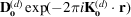; (ii) a partially reflected wavefield inside the crystal.

The corresponding tiepoints are obtained by applying the usual condition of the continuity of the tangential components of wavevectors (Fig. 5.1.7.4b). If the crystal is a plane-parallel slab, these points are M and, respectively, and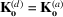.

The boundary conditions are then written:

 (i) entrance surface: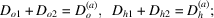(ii) back surface: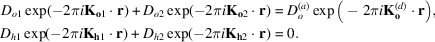Rocking curve. Using (5.1.3.10), it can be shown that the expressions for the intensities reflected at the entrance surface and transmitted at the back surface,and, respectively, are given by different expressions within total reflection and outside it:

 (i)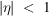: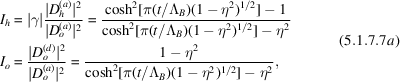where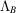is the value taken by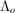[equation (5.1.3.8)] in the Bragg case. There is no longer a total-reflection domain but the extinction effect still exists, as is shown by the hyperbolic cosine term. The maximum height of the rocking curve decreases as the thickness of the crystal decreases. (ii)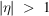: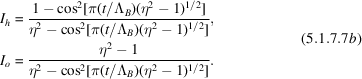The cosine terms show that the two wavefields propagating within the crystal interfere, giving rise to Pendellösung fringes in the rocking curve. These fringes were observed for the first time by Batterman & Hildebrandt (1967, 1968). The angular positions of the minima of the reflected beam are given by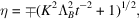where K is an integer.

Integrated intensity. The integrated intensity is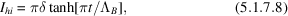where t is the crystal thickness. When this thickness becomes very large, the integrated intensity tends towards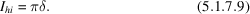This expression differs from (5.1.7.3)by the factor π, which appears here in place of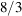. von Laue (1960)pointed out that because of the differences between the two expressions for the reflecting power, (5.1.7.2)and (5.1.7.7b), perfect agreement could not be expected. Since some absorption is always present, expression (5.1.7.3), which includes the factor, should be used for very thick crystals. In the presence of absorption, however, expression (5.1.7.8)for the reflected intensity for thin crystals does tend towards that for thick crystals as the crystal thickness increases.

Comparison with geometrical theory. When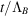is very small (thin crystals or weak reflections), (5.1.7.8)tends towards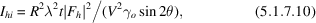which is the expression given by geometrical theory. If we call this intensity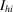(geom.), comparison of expressions (5.1.7.8)and (5.1.7.10)shows that the integrated intensity for crystals of intermediate thickness can be written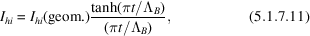which is the expression given by Darwin (1922)for primary extinction.

#### 5.1.7.2.2. Absorbing crystals

| top | pdf |

Reflected intensity. The intensity of the reflected wave for a thin absorbing crystal is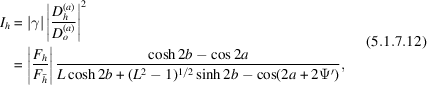where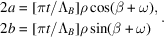L, ρ and ψ′ are defined in (5.1.7.5), β is defined in (5.1.3.7)and ω is the phase angle of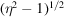.

Comparison with geometrical theory. Whendecreases towards zero, expression (5.1.7.12)tends towards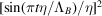; using (5.1.3.5)and (5.1.3.8), it can be shown that expression (5.1.7.12)can be written, in the non-absorbing symmetric case, as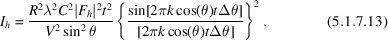where d is the lattice spacing and Δθ is the difference between the angle of incidence and the middle of the reflection domain. This expression is the classical expression given by geometrical theory [see, for instance, James (1950)].

### References

Batterman, B. W. & Hildebrandt, G. (1967). Observation of X-ray Pendellösung fringes in Darwin reflection. Phys. Status Solidi, 23, K147–K149.Google Scholar
Batterman, B. W. & Hildebrandt, G. (1968). X-ray Pendellösung fringes in Darwin reflection. Acta Cryst. A24, 150–157.Google Scholar
Darwin, C. G. (1922). The reflection of X-rays from imperfect crystals. Philos. Mag. 43, 800–829.Google Scholar
James, R. W. (1950). The optical principles of the diffraction of X-rays. London: G. Bell and Sons Ltd.Google Scholar
Laue, M. von (1960). Röntgenstrahl-Interferenzen. Frankfurt am Main: Akademische Verlagsgesellschaft.Google Scholar